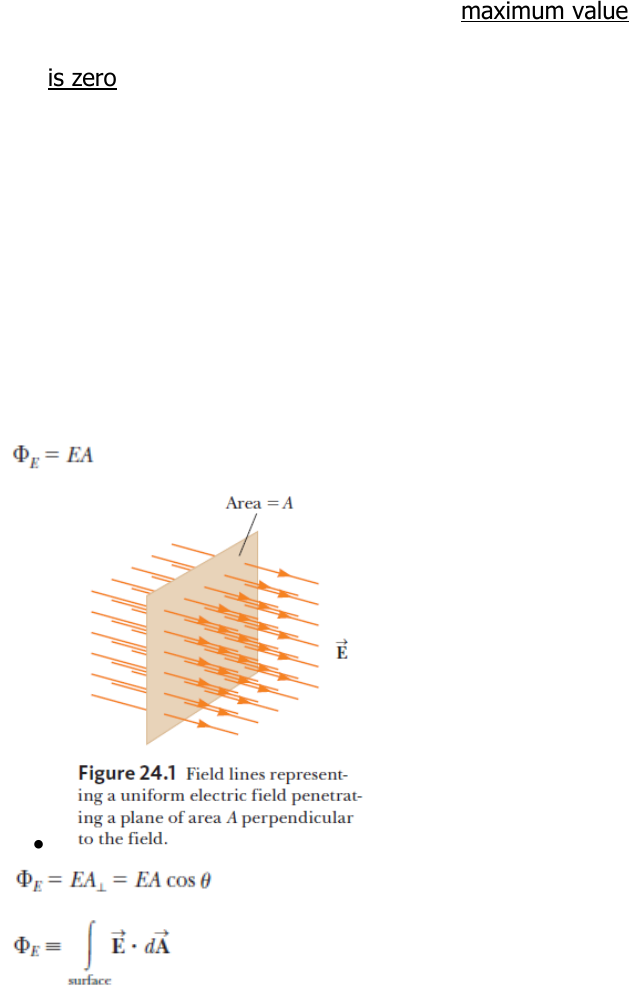Textbook Notes (280,000)
CA (170,000)
McGill (5,000)
Physics (60)
Chapter 24

# PHYS 142 Chapter Notes - Chapter 24: Electric Flux, Electric Field, Surface Charge

Department
Physics
Course Code
PHYS 142
Professor
Yoichi Miyahara
Chapter
24

Page:
of 6PHYS 142 Chapter 24 Smart Notes
24.1: Electric Flux
o Definition
The product of the electric field
E
and surface area
A
perpendicular
to the field is called the electric flux.
Electric flux is proportional to the number of electric field lines
penetrating some surface.
Flux though a surface of fixed area A has a maximum value EA
when the surface is perpendicular to the field, when  
Flux is zero when the surface is parallel to the field when the
normal to the surface is perpendicular to the field, when 
The net flux through the surface is proportional to the net number
of lines leaving the surface, where the net number means the
number of lines leaving the surface minus the number of lines
entering the surface.
If more lines are leaving than entering, then net flux is
positive.
If more lines are entering than leaving, then net flux is
negative.
o Equation
o SI Units
24.2: Gauss’s Law
Net Electric Flux in Spherical Surface
Substitute:
o The net flux through any closed surface surrounding a point charge
q
is
given by and is independent of the shape of that surface.
o The net electric flux though a closed surface that surrounds no charge is
zero.